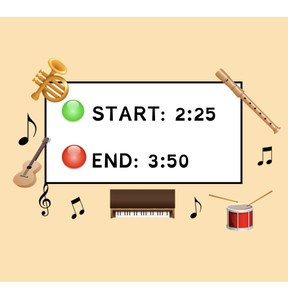Time difference: Digital clocks with 10 and 5 minutes

# Time difference: Digital clocks with 10 and 5 minutes

I can determine time differences between digital clocks with 10 and 5 minutes.8,000 schools use Gynzy92,000 teachers use Gynzy1,600,000 students use Gynzy

## General

In this lesson, students will practice calculating time difference and elapsed time between digital clocks with 10 and 5 minutes. Students will be given the skills to find the time that has passed between two events. Students will learn the “Mountain Method.” Students complete several activities in which they practice calculating elapsed time.

## Standards

CCSS.Math.Content.3.MD.A.1

## Learning Objective

Students will be able to properly tell what the difference is between two digital clocks with 10 and 5 minutes using the Mountain Method.

## Introduction

Students will review the concept of elapsed time and then answer a set of true or false questions using what they know about elapsed time and digital clocks.

## Instruction

The lesson will begin with students learning about how to utilize the “Mountain Method” to calculate elapsed time. Guide students in learning and understanding the 4 steps and what increment of time each symbol represents. Then, students will be instructed on how to utilize the method in a guided example, followed by another word problem they will solve on their own by dragging the mountains, hills, and rocks and adjusting each clock. In addition, students will watch a video in which they will learn all about calculating elapsed time with the “Mountain Method” and then create an elapsed time word problem of their own. Students will practice by completing several activities such as filling in the elapsed time for a train schedule.

## Quiz

Students respond to ten multiple-choice and true/false questions. They will read word problems and determine the correct elapsed time.

## Closing

Students look at a train schedule and determine the time elapsed for each train ride.

### The online teaching platform for interactive whiteboards and displays in schools

• Save time building lessons

• Manage the classroom more efficiently

• Increase student engagement